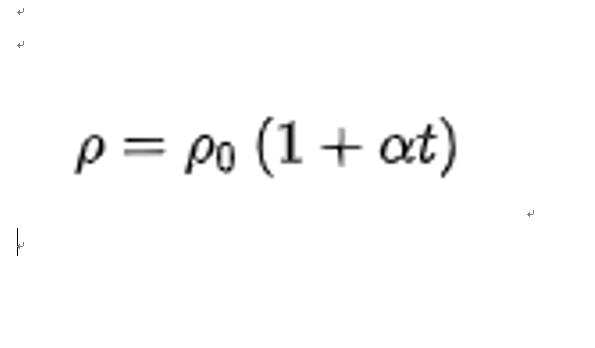# What is resistance? What are the factors that affect resistance?4

### What is resistance?

The resistance of a conductor to an electric current is called the resistance of that conductor. Resistor (usually denoted by “R”) is a physical quantity that in physics indicates the amount of resistance of a conductor to current. The higher the resistance of a conductor, the greater the resistance of the conductor to current. The resistance is generally different for different conductors and is a property of the conductor itself. The resistance of a conductor is usually expressed by the letter R. The unit of resistance is Ohm, abbreviated as Ohm, and the symbol is

### What is the function of the series resistor of the output of the active crystal?### What is a resistor? What are the parts of a resistor?